### Statistics Class 9th Mathematics Term 2 Tamilnadu Board Solution

##### Question 1.| The mean of the first 10 natural numbers isA. 25B. 55C. 5.5D. 2.5Answer:Let us list out first 10 natural numbers. They are:1, 2, 3, 4, 5, 6, 7, 8, 9, 10Total number of observations = 10Mean is given by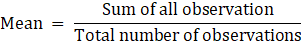⇒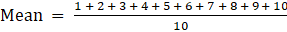⇒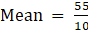⇒ Mean = 5.5Thus, option (C) is correct.Question 2.The Arithmetic mean of integers from-5 to 5 isA. 3B. 0C. 25D. 10Answer:The integers from -5 and 5 are:-5, -4, -3, -2, -1, 0, 1, 2, 3, 4, 5Total number of observations = 11Mean is given by⇒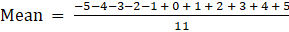⇒ Mean = 0Thus, option (B) is correct.Question 3.If the mean of x,x + 2,x + 4,x + 6,x + 8 is 20 then x isA. 32B. 16C. 8D. 4Answer:Here, total number of observations = 5And given that, mean = 20Mean is given by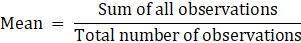⇒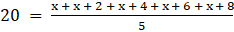⇒ 5x + 20 = 5 × 20⇒ 5x + 20 = 100⇒ 5x = 100 – 20⇒ 5x = 80⇒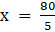⇒ x = 16Thus, option (B) is correct.Question 4.The mode of the data 5,5,5,5,5,1,2,2,3,3,3,4,4,4,4 isA. 2B. 3C. 4D. 5Answer:We can arrange the data in ascending form as:1, 2, 2, 3, 3, 3, 4, 4, 4, 4, 5, 5, 5, 5, 5This gives us an ease of observation.Here, 5 is coming 5 times and it is the most occurring value in the data.Since, mode is a number having the highest frequency of all numbers in the data.⇒ Mode = 5Thus, option (D) is correct.Question 5.The median of 14, 12, 10,9,11 isA. 11B. 10C. 9.5D. 10.5Answer:To find median, we need to arrange data in ascending order.We have9, 10, 11 ,12, 14Total number of observations, n = 5 (odd).When n is odd, median is found as follows.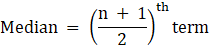⇒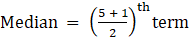⇒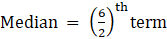⇒ Median = 3rd term⇒ Median = 11 [∵ the 3rd term in the arranged data = 11]Thus, option (A) is correct.Question 6.The median of 2,7,4,8,9,1 isA. 4B. 6C. 5.5D. 7Answer:To find median, we need to arrange data in ascending order.We have1, 2, 4, 7, 8, 9Total number of observations, n = 6 (even).When n is even, median is found as follows.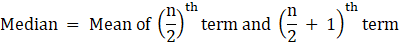⇒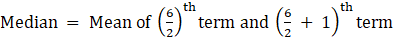⇒ Median = Mean of 3rd term and 4th term⇒ Median = Mean of 4 and 7 [∵ from the arranged data, 3rd term = 4 and 4th term = 7]⇒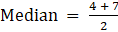⇒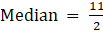⇒ Median = 5.5Thus, option (C) is correct.Question 7.The mean of first 5 whole number isA. 2B. 2.5C. 3D. 0Answer:The first whole numbers are: 0, 1, 2, 3, 4Total number of observations = 5Mean is given by⇒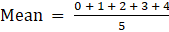⇒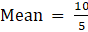⇒ Mean = 2Thus, option (A) is correct.Question 8.The arithmetic mean of 10 number is-7. If 5 is added to every number, then the new Arithmetic mean isA. -2B. 12C. -7D. 17Answer:Given that, arithmetic mean of 10 numbers = -7We know, total number of observations, n = 10Then, mean is given by⇒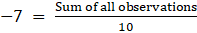⇒ Sum of all observations = 10 × -7 = -70According to question, if 5 is added to every 10 number then,New sum of all observations = Old sum + (5 × 10)⇒ New sum = -70 + 50 = -20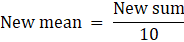⇒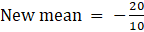⇒ New mean = -2Thus, option (A) is correct.Question 9.The Arithmetic mean of all the factors of 24 isA. 8.5B. 5.67C. 7D. 7.5Answer:All the factors of 24 are 1, 2, 3, 4, 6, 8, 12, 24. [These numbers divide 24 completely]Total number of observations = 8Mean is given by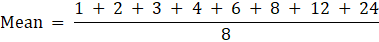⇒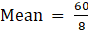⇒ Mean = 7.5Thus, option (D) is correct.Question 10.The mean of 5 numbers is 20. If one number is excluded their mean is 15. Then the excluded number isA. 5B. 40C. 20D. 10Answer:Given that, Total number of observations = 5Mean = 20So, Sum of observations can be given asSum of observations = Mean × Total number of observations [∵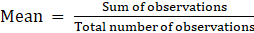]⇒ Sum of observations = 20 × 5⇒ Sum of observations = 100Let the excluded number be x.New Sum of observations = 100 – xNew Mean = 15New total number of observations = 4Mean is given by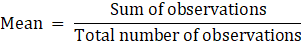⇒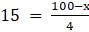⇒ 100 – x = 15 × 4⇒ 100 – x = 60⇒ x = 100 – 60 = 40⇒ The excluded number is 40.Thus, option (B) is correct.

PDF FILE TO YOUR EMAIL IMMEDIATELY PURCHASE NOTES & PAPER SOLUTION. @ Rs. 50/- each (GST extra)

HINDI ENTIRE PAPER SOLUTION

MARATHI PAPER SOLUTION

SSC MATHS I PAPER SOLUTION

SSC MATHS II PAPER SOLUTION

SSC SCIENCE I PAPER SOLUTION

SSC SCIENCE II PAPER SOLUTION

SSC ENGLISH PAPER SOLUTION

SSC & HSC ENGLISH WRITING SKILL

HSC ACCOUNTS NOTES

HSC OCM NOTES

HSC ECONOMICS NOTES

HSC SECRETARIAL PRACTICE NOTES

# 2019 Board Paper Solution

HSC ENGLISH SET A 2019 21st February, 2019

HSC ENGLISH SET B 2019 21st February, 2019

HSC ENGLISH SET C 2019 21st February, 2019

HSC ENGLISH SET D 2019 21st February, 2019

SECRETARIAL PRACTICE (S.P) 2019 25th February, 2019

HSC XII PHYSICS 2019 25th February, 2019

CHEMISTRY XII HSC SOLUTION 27th, February, 2019

OCM PAPER SOLUTION 2019 27th, February, 2019

HSC MATHS PAPER SOLUTION COMMERCE, 2nd March, 2019

HSC MATHS PAPER SOLUTION SCIENCE 2nd, March, 2019

SSC ENGLISH STD 10 5TH MARCH, 2019.

HSC XII ACCOUNTS 2019 6th March, 2019

HSC XII BIOLOGY 2019 6TH March, 2019

HSC XII ECONOMICS 9Th March 2019

SSC Maths I March 2019 Solution 10th Standard11th, March, 2019

SSC MATHS II MARCH 2019 SOLUTION 10TH STD.13th March, 2019

SSC SCIENCE I MARCH 2019 SOLUTION 10TH STD. 15th March, 2019.

SSC SCIENCE II MARCH 2019 SOLUTION 10TH STD. 18th March, 2019.

SSC SOCIAL SCIENCE I MARCH 2019 SOLUTION20th March, 2019

SSC SOCIAL SCIENCE II MARCH 2019 SOLUTION, 22nd March, 2019

XII CBSE - BOARD - MARCH - 2019 ENGLISH - QP + SOLUTIONS, 2nd March, 2019

# HSCMaharashtraBoardPapers2020

(Std 12th English Medium)

HSC ECONOMICS MARCH 2020

HSC OCM MARCH 2020

HSC ACCOUNTS MARCH 2020

HSC S.P. MARCH 2020

HSC ENGLISH MARCH 2020

HSC HINDI MARCH 2020

HSC MARATHI MARCH 2020

HSC MATHS MARCH 2020

# SSCMaharashtraBoardPapers2020

(Std 10th English Medium)

English MARCH 2020

HindI MARCH 2020

Hindi (Composite) MARCH 2020

Marathi MARCH 2020

Mathematics (Paper 1) MARCH 2020

Mathematics (Paper 2) MARCH 2020

Sanskrit MARCH 2020

Sanskrit (Composite) MARCH 2020

Science (Paper 1) MARCH 2020

Science (Paper 2)

Geography Model Set 1 2020-2021

MUST REMEMBER THINGS on the day of Exam

Are you prepared? for English Grammar in Board Exam.

Paper Presentation In Board Exam

How to Score Good Marks in SSC Board Exams

Tips To Score More Than 90% Marks In 12th Board Exam

How to write English exams?

How to prepare for board exam when less time is left

How to memorise what you learn for board exam

No. 1 Simple Hack, you can try out, in preparing for Board Exam

How to Study for CBSE Class 10 Board Exams Subject Wise Tips?

JEE Main 2020 Registration Process – Exam Pattern & Important Dates

NEET UG 2020 Registration Process Exam Pattern & Important Dates

How can One Prepare for two Competitive Exams at the same time?

8 Proven Tips to Handle Anxiety before Exams!